Energy, Enthalpy, and the First Law of Thermodynamics

Energy, Enthalpy, and the First Law of Thermodynamics

Chemical Thermodynamics

Thermodynamics is defined as the branch of science that deals with the relationship between heat and other forms of energy, such as work. It is frequently summarized as three laws that describe restrictions on how different forms of energy can be interconverted. Chemical thermodynamics is the portion of thermodynamics that pertains to chemical reactions.

 The Laws of Thermodynamics First law: Energy is conserved; it can be neither created nor destroyed. Second law: In an isolated system, natural processes are spontaneous when they lead to an increase in disorder, or entropy. Third law: The entropy of a perfect crystal is zero when the temperature of the crystal is equal to absolute zero (0 K).

There have been many attempts to build a device that violates the laws of thermodynamics. All have failed. Thermodynamics is one of the few areas of science in which there are no exceptions.The System and Surroundings

One of the basic assumptions of thermodynamics is the idea that we can arbitrarily divide the universe into a system and its surroundings. The boundary between the system and its surroundings can be as real as the walls of a beaker that separates a solution from the rest of the universe (as in the figure below).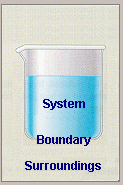Or it can be as imaginary as the set of points that divide the air just above the surface of a metal from the rest of the atmosphere (as in the figure below).Internal Energy

One of the thermodynamic properties of a system is its internal energy, E, which is the sum of the kinetic and potential energies of the particles that form the system. The internal energy of a system can be understood by examining the simplest possible system: an ideal gas. Because the particles in an ideal gas do not interact, this system has no potential energy. The internal energy of an ideal gas is therefore the sum of the kinetic energies of the particles in the gas.

The kinetic molecular theory assumes that the temperature of a gas is directly proportional to the average kinetic energy of its particles, as shown in the figure below.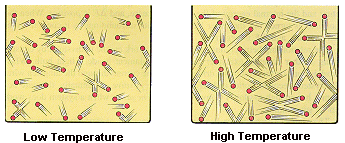The internal energy of an ideal gas is therefore directly proportional to the temperature of the gas.

Esys = 3/2 RT

In this equation, R is the ideal gas constant in joules per mole kelvin (J/mol-K) and T is the temperature in kelvin.

The internal energy of systems that are more complex than an ideal gas can't be measured directly. But the internal energy of the system is still proportional to its temperature. We can therefore monitor changes in the internal energy of a system by watching what happens to the temperature of the system. Whenever the temperature of the system increases we can conclude that the internal energy of the system has also increased.

Assume, for the moment, that a thermometer immersed in a beaker of water on a hot plate reads 73.5oC, as shown in the figure below. This measurement can only describe the state of the system at that moment in time. It can't tell us whether the water was heated directly from room temperature to 73.5oC or heated from room temperature to 100oC and then allowed to cool.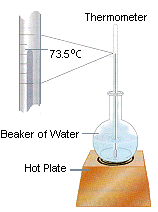Temperature is therefore a state function. It depends only on the state of the system at any moment in time, not the path used to get the system to that state. Because the internal energy of the system is proportional to its temperature, internal energy is also a state function. Any change in the internal energy of the system is equal to the difference between its initial and final values.Esys = Ef - EiThe First Law Of Thermodynamics

The first law of thermodynamics can be captured in the following equation, which states that the energy of the universe is constant. Energy can be transferred from the system to its surroundings, or vice versa, but it can't be created or destroyed.

 First Law of Thermodynamics:Euniv =Esys +Esurr = 0

A more useful form of the first law describes how energy is conserved. It says that the change in the internal energy of a system is equal to the sum of the heat gained or lost by the system and the work done by or on the system.

 First Law of Thermodynamics:Esys = q + w

The sign convention for the relationship between the internal energy of a system and the heat gained or lost by the system can be understood by thinking about a concrete example, such as a beaker of water on a hot plate. When the hot plate is turned on, the system gains heat from its surroundings. As a result, both the temperature and the internal energy of the system increase, andE is positive. When the hot plate is turned off, the water loses heat to its surroundings as it cools to room temperature, andE is negative.

The relationship between internal energy and work can be understood by considering another concrete example: the tungsten filament inside a light bulb. When work is done on this system by driving an electric current through the tungsten wire, the system becomes hotter andE is therefore positive. (Eventually, the wire becomes hot enough to glow.) Conversely,E is negative when the system does work on its surroundings.

The sign conventions for heat, work, and internal energy are summarized in the figure below. The internal energy and temperature of a system decrease (E < 0) when the system either loses heat or does work on its surroundings. Conversely, the internal energy and temperature increase (E > 0) when the system gains heat from its surroundings or when the surroundings do work on the system.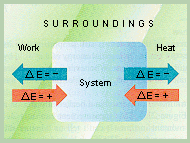The System and Work

The system is usually defined as the chemical reaction and the boundary is the container in which the reaction is run. In the course of the reaction, heat is either given off or absorbed by the system. Furthermore, the system either does work on it surroundings or has work done on it by its surroundings. Either of these interactions can affect the internal energy of the system.Esys = q + w

Two kinds of work are normally associated with a chemical reaction: electrical work and work of expansion. Chemical reactions can do work on their surroundings by driving an electric current through an external wire. Reactions also do work on their surroundings when the volume of the system expands during the course of the reaction The amount of work of expansion done by the reaction is equal to the product of the pressure against which the system expands times the change in the volume of the system.

w = - PV

The sign convention for this equation reflects the fact that the internal energy of the system decreases when the system does work on its surroundings.Enthalpy Versus Internal Energy

What would happen if we created a set of conditions under which no work is done by the system on its surroundings, or vice versa, during a chemical reaction? Under these conditions, the heat given off or absorbed by the reaction would be equal to the change in the internal energy of the system.Esys = q (if and only if w = 0)

The easiest way to achieve these conditions is to run the reaction at constant volume, where no work of expansion is possible. At constant volume, the heat given off or absorbed by the reaction is equal to the change in the internal energy that occurs during the reaction.Esys = qv (at constant volume)

The figure below shows a calorimeter in which reactions can be run at constant volume. Most reactions, however, are run in open flasks and beakers. When this is done, the volume of the system is not constant because gas can either enter or leave the container during the reaction. The system is at constant pressure, however, because the total pressure inside the container is always equal to atmospheric pressure.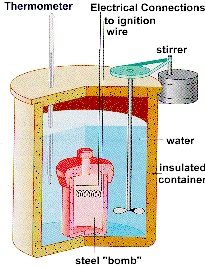If a gas is driven out of the flask during the reaction, the system does work on its surroundings. If the reaction pulls a gas into the flask, the surroundings do work on the system. We can still measure the amount of heat given off or absorbed during the reaction, but it is no longer equal to the change in the internal energy of the system, because some of the heat has been converted into work.Esys = q + w

We can get around this problem by introducing the concept of enthalpy (H), which is the sum of the internal energy of the system plus the product of the pressure of the gas in the system times the volume of the system.

Hsys = Esys + PV

For the sake of simplicity, the subscript "sys" will be left off the symbol for both the internal energy of the system and the enthalpy of the system from now on. We will therefore abbreviate the relationship between the enthalpy of the system and the internal energy of the system as follows.

H = E + PV

The change in the enthalpy of the system during a chemical reaction is equal to the change in its internal energy plus the change in the product of the pressure times the volume of the system.H =E +(PV)

Let's assume that the reaction is run in a styrofoam cup, as shown in the figure below.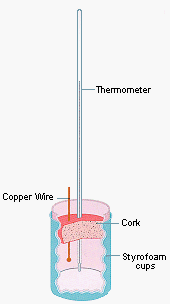Because the reaction is run at constant pressure, the change in the enthalpy that occurs during the reaction is equal to the change in the internal energy of the system plus the product of the constant pressure times the change in the volume of the system.H =E + PV (at constant pressure)

Substituting the first law of thermodynamics into this equation gives the following result.H = (qp + w) + PV

Assuming that the only work done by the reaction is work of expansion gives an equation in which the PV terms cancel.H = (qp - PV) + PV

Thus, the heat given off or absorbed during a chemical reaction at constant pressure is equal to the change in the enthalpy of the system.H = qp (at constant pressure)

The relationship between the change in the internal energy of the system during a chemical reaction and the enthalpy of reaction can be summarized as follows.

1. The heat given off or absorbed when a reaction is run at constant volume is equal to the change in the internal energy of the system.Esys = qv

2. The heat given off or absorbed when a reaction is run at constant pressure is equal to the change in the enthalpy of the system.Hsys = qp

3. The change in the enthalpy of the system during a chemical reaction is equal to the change in the internal energy plus the change in the product of the pressure of the gas in the system and its volume.Hsys =Esys +(PV)

4. The difference betweenE andH for the system is small for reactions that involve only liquids and solids because there is little if any change in the volume of the system during the reaction. The difference can be relatively large, however, for reactions that involve gases, if there is a change in the number of moles of gas in the course of the reaction.

 Practice Problem 1:Which of the following processes are run at constant volume and which are run at constant pressure? (a) an acid-base titration (b) decomposing CaCo3 by heating limestone in a crucible with a bunsen burner (c) the reaction bewteen zinc metal and an aqueous solution of Cu2+ ions to form copper metal and Zn2+ ions (d) measuring the calories in a 1-oz. serving of breakfast cereal by burning the cereal in a bomb calorimeter Click here to check your answer to Practice Problem 1.